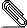# Re: [NTG-context] [luatex-plain] disappearing math-on node

···<date: 2014-04-03, Thursday>···<from: Hans Hagen>···

> On 4/3/2014 6:29 PM, Dohyun Kim wrote:
> > This is a bug report based on the issue discussed at
> >
> > \font\tenrm{file:lmroman10-regular.otf:mode=node;script=latn}\tenrm
> > \setbox0\vbox{%
> >    x\penalty-10000
> >    $a$x$a$
> > }
> > \unvbox0
> > \setbox0\lastbox
> > \unhbox0
> > \end
> >
> > The plain tex code shown above fails with a lua error:
> > luatex-fonts-merged.lua:9616: attempt to index local 'current' (a nil value)
> >
> > My guess is: as math-on (math-off too) is a "discardable" item after a
> > linebreak, the first math-on node has gone away. So the
> > math-on/math-off pair has become broken, by which the behavior of
> > "node.end_of_math" is confused.
>
> mtxrun --script plain test.tex
>
> runs ok here so i need an example that fails with context or luatex-plain

It fails with the luatex-plain format from the minimals as of
yesterday and Luatex 4972:

error: .../context/tex/texmf-context/tex/context/base/font-ota.lua:144:
attempt to index local 'current' (a nil value)
.
\bye ^^@-\par
\vfill \supereject \end
l.17 \bye

Though the issue arises only in node mode.

> normally the font callback is called on a hlist and there are always
> paired math nodes
>
> (what is line 9616 in the current beta?)

Line 9626 with yesterday’s code. Attached are patches for
font-ota.lua and luatex-fonts-otn.lua that guard all invocations
of end_of_math() with checks for the subtype.

Best,
Philipp


--- luatex-fonts-otn.lua.orig	2014-04-05 15:02:34.711828352 +0200
+++ luatex-fonts-otn.lua	2014-04-05 15:02:36.955171990 +0200
@@ -2375,7 +2375,7 @@
end
end
start = start.next
-                        elseif id == math_code then
+                        elseif id == math_code and start.subtype == 0 then
start = end_of_math(start).next
else
start = start.next
@@ -2567,7 +2567,7 @@
end
end
start = start.next
-                    elseif id == math_code then
+                    elseif id == math_code and start.subtype == 0 then
start = end_of_math(start).next
else
start = start.next

--- font-ota.lua.orig	2014-04-05 14:55:53.789969704 +0200
+++ font-ota.lua	2014-04-05 14:59:32.487654509 +0200
@@ -137,7 +137,7 @@
last[a_state] = s_fina
end
first, last, n = nil, nil, 0
-            if id == math_code then
+            if id == math_code and current.subtype == 0 then
current = end_of_math(current)
end
end
@@ -423,7 +423,7 @@
if first or last then
first, last = finish(first,last)
end
-            if id == math_code then
+            if id == math_code and current.subtype == 0 then
current = end_of_math(current)
end
endpgpCSlyjCsqyV.pgp
Description: PGP signature

___________________________________________________________________________________
___________________________________________________________________________________